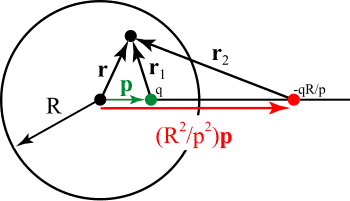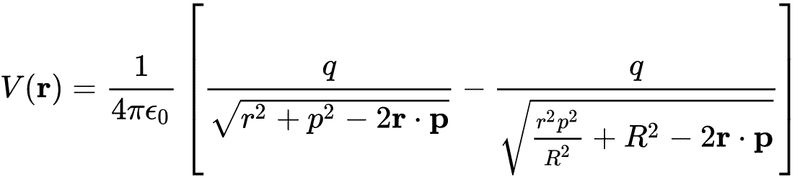# Potential outside a grounded conductor with point charge inside

daiisibil
Homework Statement:
If we have a grounded sphere with a point charge inside, what is the potential outside?
What if it's not a sphere and arbitrary shape?
Relevant Equations:
Poisson equation for electrostatic potential
Potential inside is given as in ,https://en.wikipedia.org/wiki/Method_of_image_charges, which is the sum of excitation and induced potential. When the charge is outside it is easy to argue potential is zero in the sphere. But when we have charge inside and image outside, what is potential outside?1) could one use a Gaussian surface enclosing the charge q and surface charge density?
2) in the case of a sphere it's easy to integrate surface charge, what about asymmetric volume?
PS apologies for some reason couldn't write in tex.

•Delta2

Gold Member
Charge -q is supplied from the ground Earth to the sphere and it together with point charge q cancel out electric field outside to make V=0. Not only sphere but any shape is all right for this cancellation outside.

•daiisibil and baby_1
baby_1
Homework Statement:: If we have a grounded sphere with a point charge inside, what is the potential outside?
What if it's not a sphere and arbitrary shape?
Relevant Equations:: Poisson equation for electrostatic potential

Potential inside is given as in ,https://en.wikipedia.org/wiki/Method_of_image_charges, which is the sum of excitation and induced potential. When the charge is outside it is easy to argue potential is zero in the sphere. But when we have charge inside and image outside, what is potential outside?
View attachment 286626View attachment 286628

1) could one use a Gaussian surface enclosing the charge q and surface charge density?
2) in the case of a sphere it's easy to integrate surface charge, what about asymmetric volume?
PS apologies for some reason couldn't write in tex.

Hello,
1) The Gaussian surface around the charge is a Sphere too. So we can write:
$$D_{r}4\pi r^2=q_{in}=>D_{r}=\frac{q_{in}}{\pi r^2}=>E_{r}=\frac{q_{in}}{\varepsilon \pi r^2}$$
It is valid between the Sphere and charge. Outside of sphere we have no charge regarding the sphere is grounded.
2) For complex structure we use numerical electromagnetic approaches if we are unable to calculate the integral easily.However, if the structure is PEC and is grounded the same approach -as anuttarasammyak said- can be applied.

•daiisibil and Delta2
daiisibil
Hello,
1) The Gaussian surface around the charge is a Sphere too. So we can write:
$$D_{r}4\pi r^2=q_{in}=>D_{r}=\frac{q_{in}}{\pi r^2}=>E_{r}=\frac{q_{in}}{\varepsilon \pi r^2}$$
It is valid between the Sphere and charge. Outside of sphere we have no charge regarding the sphere is grounded.
2) For complex structure we use numerical electromagnetic approaches if we are unable to calculate the integral easily.However, if the structure is PEC and is grounded the same approach -as anuttarasammyak said- can be applied.
For the charge +q inside, the induced charge on the boundary is -qR/p, so in a Gaussian surface outside shouldn't we see a charge equal to the sum of these and thus have electric field and potential difference?

baby_1
Based on the Gaussian law $qin$ is the charge which is enclosed by the surface not out of the Gaussian surface. Total charge which is the sum of +q and induced charge is equal to each other and cancel each other( when the sphere is grounded). If sphere is not grounded the the Total charge out of the sphere is +q.

•daiisibil
daiisibil
Thank for the reply, I don't know why I confused the total charge with the image point charge.
If you don't mind another rudimentary question, in the case of the sphere we find surface charge density by taking the gradient of the potential evaluated at the boundar, and to find total charge we integrate charge density. Is there an easy argument for why the total charge of an arbitrary shaped grounded conductor is equal to the charge inside it?

baby_1
Dear daiisibil,

First, It is not the image theorem when the surface is not limited. It is just a simple question:
if we have a conductor near a positive charge, based on the free electron which exist on the conductor they are affected by the charge electric field and they sit on the conductor surface. So when the electrons sit on the one side of conductor on the other side of the conductor positive charges appear. if we ground the conductor, the positive charges goes to the ground.

•daiisibil
baby_1
•daiisibil